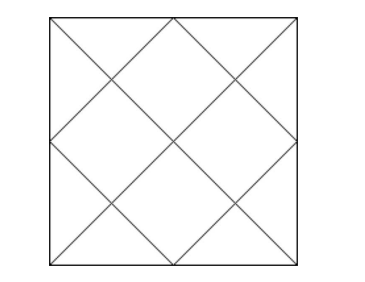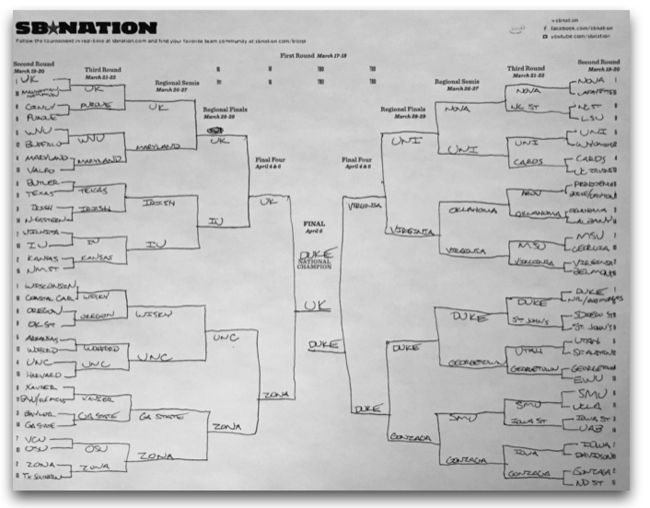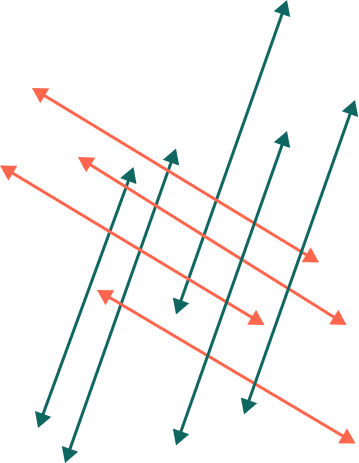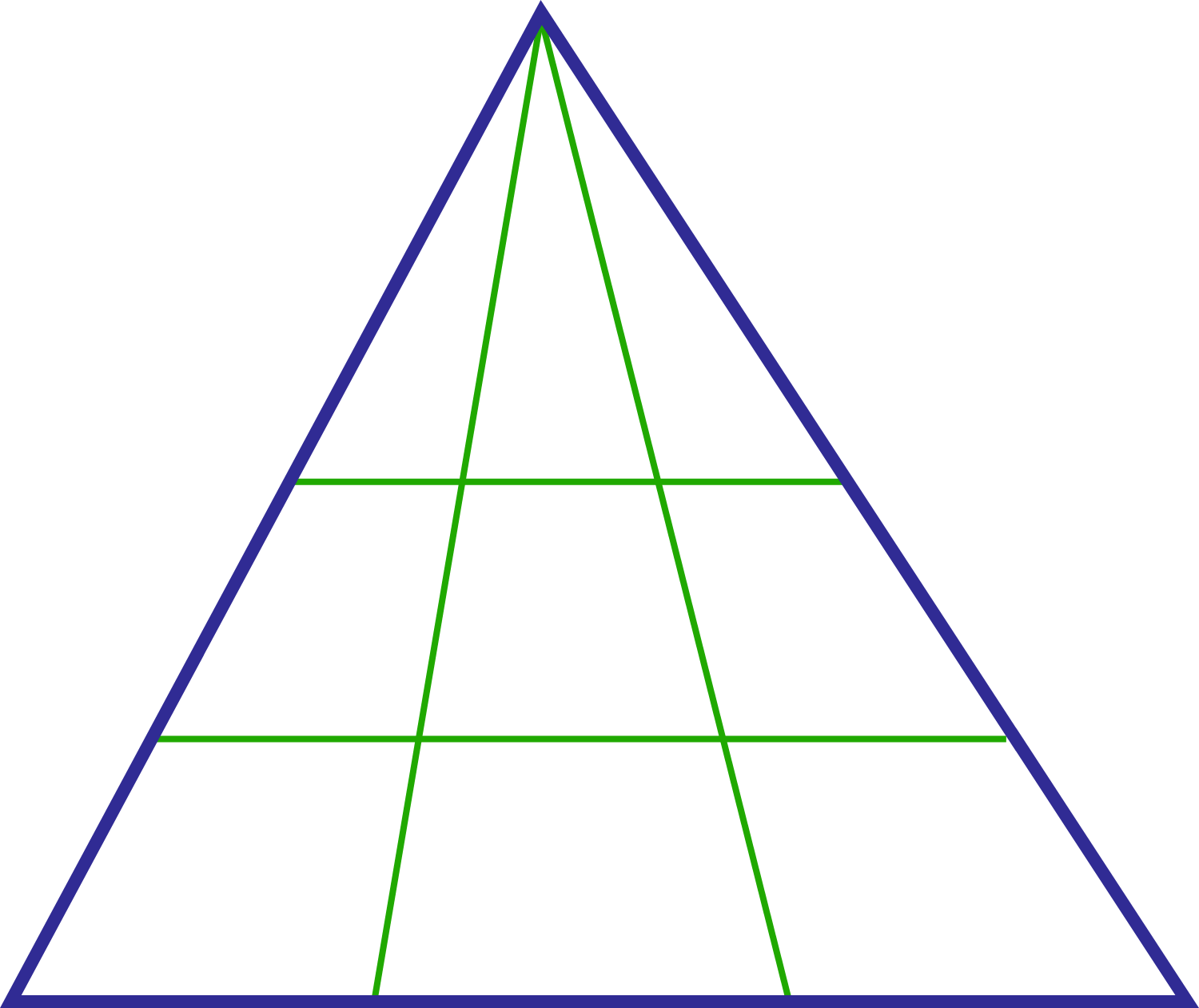Probability

# Rule of Sum and Rule of Product: Level 3 ChallengesHow many triangles are there in the diagram above?In the single-elimination NCAA Tournament, 64 teams compete in 6 bracketed rounds. How many games are there in total?How many 4 -digit positive integers have 2 or more zeroes?How many parallelograms are formed when a set of 5 parallel lines intersects a set of 4 parallel lines?

Details and Assumptions

• All parallel lines are extended indefinitely.How many triangles are there in the above image?

×